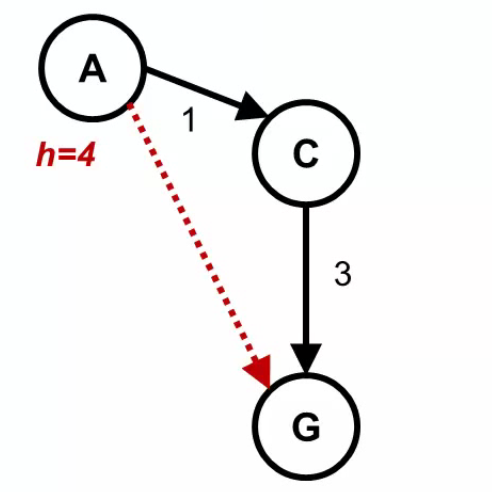# Can someone give me an example of an admissible heuristic that is not consistent?

742    Asked by bruce_8968 in Python , Asked on Jul 5, 2021

In this figure:let's assume that h(C)=1 If f(A)=g(A)+h(A)=0+4=4, and f(C)=g(C)+h(C)=1+1=2 Then f(C) is NOT greater than or equal to f(A) Therefore this example is consistent and admissible, but can someone give me an admissible heuristic  example that is not consistent? please

CONSISTENT HEURISTIC:

A heuristic in the algorithm is consistent if the cost from the current node to a successor node, plus the estimated cost from the successor node to the goal is less than or equal to the estimated cost from the current node to the goal.

In an equation, it would look like this: C(n, n’) + h(n’) ≤ h(n)

A heuristic function is admissible if the estimated cost is never more than the actual cost from the current node to the goal node.

If you want inconsistency and since h(C) <= 3 for the admissibility condition then you should have that h(A) > 1 + h(C). So any heristics that satisfies:

h(A) > 1 + h(C)

h(C) <= 3

h(G) = 0

is admissible and not consistent. You gave

h(A) = 4

h(C) = 1

h(G) = 0

which is a valid candidate. This is admissible heuristic example.